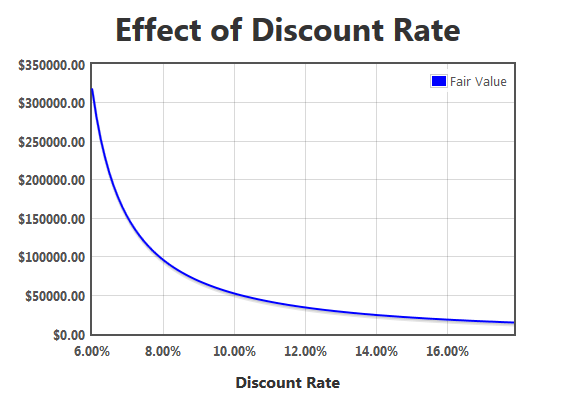Dear Valued Visitor,

We have noticed that you are using an ad blocker software.

Although advertisements on the web pages may degrade your experience, our business certainly depends on them and we can only keep providing you high-quality research based articles as long as we can display ads on our pages.

# Facebook Inc (FB), Tesla Motors Inc (TSLA): Be Careful With Discounted Cash Flows

Page 1 of 2

The most fundamental way in which to value a stock is by performing a discounted cash flow calculation, or DCF. If you’re a regular reader of articles about stocks you’ve likely seen this method used countless times. DCF calculations are ultra-sensitive to the inputs, meaning that a small change in a parameter can lead to a dramatic change in the result. This is dangerous if you blindly trust a calculation, as many seem to do. It’s important to understand exactly what can go wrong.A quick overview

The value of a company is the sum of all future cash flows discounted back to today by a discount rate, plus or minus any net cash or net debt. Imagine a company which makes \$1 million in profit every year and expects to continue to do so into the foreseeable future. If we use a discount rate of 10% then next year’s profit is worth about \$909 thousand today, the profit in the year after that is worth \$826 thousand, and so on. Adding this all up we arrive at a company value of \$10 million.

This makes sense, since if we bought the company for \$10 million today the profit would give us a 10% return on our investment, exactly our discount rate. The discount rate, then, is your required rate of return. If I had used a 12% discount rate in the example above then the value of the company would have been only \$8.33 million. An 8% discount rate would have led to a \$12.5 million valuation.

When you value a stock the process is exactly the same, except the profits are typically expected to grow over time. In general, the formula for a DCF calculation is:where r is the discount rate, C is the cash flow in each year, and i is the year. In the example above, with a constant profit, C would always be the same.

I’ll use two different stocks to point out the potential problems with DCF calculations, Facebook Inc (NASDAQ:FB) and Tesla Motors Inc (NASDAQ:TSLA).

Problem 1: The discount rate

Facebook Inc (NASDAQ:FB) recorded a free cash flow of \$377 million in 2012, and for the sake of simplicity I’ll use this value in my calculation. Let’s assume that the free cash flow grows by 30% annually for the next 10 years and by 5% annually after that. Whether this assumption is reasonable or not doesn’t really matter, because what I’m interested in is how the choice of the discount rate changes the value of the company.If you use a discount rate of 10% you arrive at a fair value of about \$52 billion, not too far off from the current market capitalization. If you bump that up to 15%, a value which I like to use, the fair value plummets to just \$21 billion.

Here’s where it gets dangerous. I’ve seen people use discount rates around 7% when dealing with companies like Facebook Inc (NASDAQ:FB). This would lead to a fair value of \$150 billion! That’s a factor of 7 higher than the value with a 15% discount rate.

Which discount rate is correct? None of them are. The discount rate is your required rate of return, and that varies from person to person. I like to use both 12% and 15% to get a nice fair value range, implying that I want my rate of return to be between these values. Using a 7% discount rate assumes that you only want a 7% return, in which case you should just go buy an broad-market ETF and not think about individual stocks anymore.

The bottom line about discount rates is this: small changes in the discount rate lead to big changes in the fair value. Too low of a discount rate will give you an unrealistically high result.

Problem 2: Growth rate is too close to the discount rate

I’ll stick with Facebook Inc (NASDAQ:FB) to demonstrate the second problem. Let’s do the same calculation as above, using a 7% discount rate, but instead of a 5% long term growth rate after 10 years let’s bump that number up to 6.5%. Surely this slight increase can’t have too big of an effect on the value of the company.

Well, it does. The fair value nearly quadruples to \$575 billion. A long-term growth rate of 6.9% values Facebook Inc (NASDAQ:FB) at \$2.8 trillion! How can such a small change lead to such outrageous values? Because the growth rate is too close to the discount rate. The fair value goes to infinity at a long-term growth rate equal to the discount rate, so any where close to that leads to huge values.

This is why you simply can’t assume that the cash flow will grow at 20% per year forever – you’d get a nonsense answer. The typical solution is choosing a fairly low long-term growth rate, say 3%, and admit that you have no idea what is actually going to happen ten years from now anyway. This brings the answers back into reality.

Problem 3: Overly optimistic growth rate

Now let’s turn to Tesla Motors Inc (NASDAQ:TSLA). Is it possible to justify Tesla’s crazy-high P/E ratio by tweaking parameters in a DCF calculation? Well, that’s likely what analysts with their price targets do every day. Tesla Motors Inc (NASDAQ:TSLA) recently had its first quarter of profitability after burning through cash for many years. The company is valued at \$12.5 billion.

Page 1 of 2